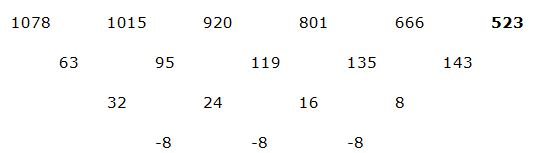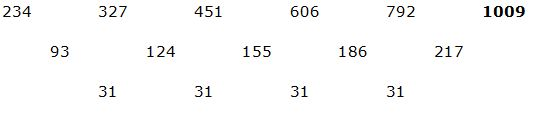# LIC HFL Assistant Manager Quantitative Aptitude Questions – (Day-02)

Dear Aspirants, Our IBPS Guide team is providing new series of Quantitative Aptitude Questions for LIC HFL Assistant Manager 2019 so the aspirants can practice it on a daily basis. These questions are framed by our skilled experts after understanding your needs thoroughly. Aspirants can practice these new series questions daily to familiarize with the exact exam pattern and make your preparation effective.

Check here for LIC HFL Mock Test 2019

Check here for IBPS RRB PO Mains Mock Test 2019

Check here for IBPS RRB Clerk Mains Mock Test 2019

### Click Here to Subscribe Crack High Level Puzzles & Seating Arrangement Questions PDF 2019 Plan

[WpProQuiz 6929]

Directions (1 – 5): Study the following information carefully and answer the given questions:

The given bar graph shows the rate of interest per annum offered by 4 different banks for deposits under three different schemes (A, B and C).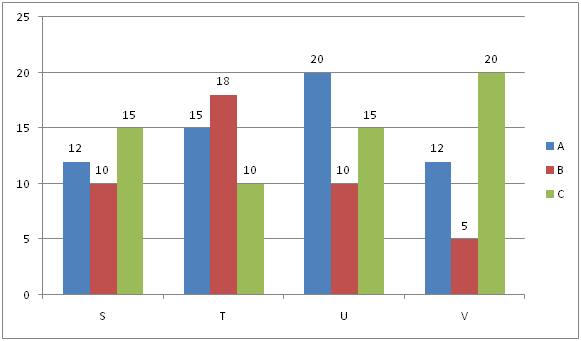1) Ram deposited an amount in scheme A of company S for two years and he also invests the same amount in scheme C of company T for two years. If the total amount of simple interest accrued from the two schemes is Rs.880, then what is the principal amount?

a) Rs.2000

b) Rs.4000

c) Rs.1500

d) Rs.3000

e) None of these

2) The company U offers compound interest under scheme B and company V offer simple interest under scheme B. What is the difference between the interests earned under these schemes in two years, if the principal amount is Rs.8400?

a) Rs.924

b) Rs.876

c) Rs.780

d) Rs.862

e) None of these

3) Sunil invested his amount Rs.4500 for eight years with company T under scheme B which is offering simple interest and Prabakar invested Rs.6000 for three years with company V under scheme C which is offering compound interest. What is the difference between the interest earned by Sunil and Prabakar?

a) Rs.2450

b) Rs.1890

c) Rs.3156

d) Rs.2789

e) None of these

4) Kavin invested Rs.3000 in company S under scheme C which is offering simple interest for 5 years and Rs.2000 in company V under scheme A which is also offering simple interest for 4 years, What is the total interest earned by Kavin?

a) Rs.2180

b) Rs.2820

c) Rs.3210

d) Rs.3840

e) None of these

5) The company T offers compound interest under scheme C and company U offer simple interest under scheme B. What is the difference between the interests earned under these schemes in three years, if the principal amount is Rs.4800?

a) Rs.152.5

b) Rs.124.8

c) Rs.148.8

d) Rs.162.4

e) None of these

Directions (6 – 10): What value should come in the place of question mark (?) in the following number series?

6) 21, ? , 39, 183, 327

a) 20

b) 25

c) 35

d) 15

e) 30

7) 17, 23, ?, 78, 183

a) 30

b) 37

c) 39

d) 47

e) 49

8) 5015, 5266, ?, 5714, 5899

a) 5501

b) 5400

c) 5611

d) 5701

e) 5500

9)  1078, 1015, 920, 801, 666, ?

a) 623

b) 614

c) 554

d) 523

e) 514

10) 234, 327, 451, 606, 792, ?

a) 1019

b) 901

c) 1009

d) 999

e) 1001

Direction (1-5) :

x * 12 * 2/100 + x * 10 * 2/100 = 880

24x + 20x = 88000

x = Rs. 2000

SI = 5 * 8400 * 2/100

SI = Rs. 840

CI = 8400 * (1 + 10/100)2 – 8400

CI = Rs. 1764

Required Difference = 1764 – 840 = Rs. 924

Interest amount earned by Sunil = 4500 * 18 * 8/100 = Rs. 6480

Interest amount earned by Prabakar = 6000 * (1 + 20/100)3 – 6000

= Rs. 4368

Required Difference = 6480 – 4368 = Rs. 2112

Total interest = 3000 * 15 * 5/100 + 2000 * 12 * 4/100

= 2250 + 960

= Rs. 3210

Difference = P * r * r/100 * 100 * 100 * (300 + r)

= 4800 * 10 * 10/100 * 100 * 100 * (300 + 10)

= 48 * 310/100

= Rs. 148.8

Direction (6-10) :

21 * (1 + 2)2 = 30

30 *(3 + 0)2 = 39

39 * (3 + 9)2 = 183

183 * (1 + 8 + 3)2 = 327

712 – (33 – 1) = 5015

732 – (43 – 1) = 5266

752 – (53 – 1) = 5501

772 – (63 – 1) = 5714

792 – (73 – 1) = 5899

(Or)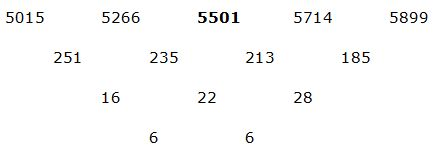712 – (33 – 1) = 5015

732 – (43 – 1) = 5266

752 – (53 – 1) = 5501

772 – (63 – 1) = 5714

792 – (73 – 1) = 5899

(Or)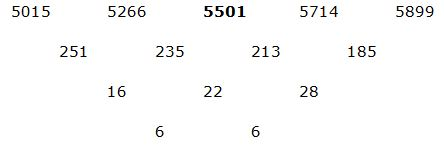1078 – (21 * 3) = 1015

1015 – (19 * 5) = 920

920 – (17 * 7) = 801

801 – (15 * 9) = 666

666 – (13 * 11) = 523

(Or)